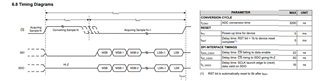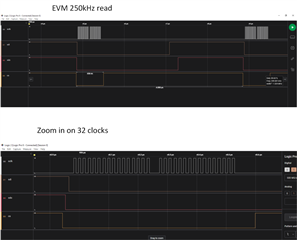If you have a related question, please click the "Ask a related question" button in the top right corner. The newly created question will be automatically linked to this question.

Hi,

I have a question regarding ADS7066.
I connected the evaluation board of this ADC, when I wanted to have a maximal sample rate of 250ksps, the SCLK couldn't be lower than 60MHz.
I need to isolate my SPI interface with opto isolators, so I have the limitation of 25MHz maximum for the SCLK signal.
my question is, why do I need 240 clock cycles per sample?
can I use a slower SCLK for my application?
where can I see the minimal SCLK for 250KSPS?

thank you very much,

Roee Kimchi

• Roee,

Below is an explanation of the clock timing requirements.  The EVM clocks out the data with 32 clocks, but only 24 are required, so it is possible to run at a somewhat lower clock rate.  However, for a sampling rate of 250kHz, you will not be able to use a 25MHz SCLK signal.  The main factor that is causing the requirement for the higher clock rate is the fact that you must keep CS high during the conversion cycle.  The maximum conversion cycle is specified at 3200ns, so you need to keep CS high for that length of time.  This only leaves 800ns for clocking out the data.  I will say that a small change in sampling rate will give quite a bit more flexibility in setting clock frequency.  For example, if you reduce the sampling rate to 200kHz, then you have 1800ns for clocking out the data (1/200kHz - 3200ns = 1800ns).  Assuming 24.5 clocks you can use a clock frequency of 13.6MHz.  Furthermore, this will make the acquisition period much longer (1800ns) which will make settling easier (this helps reduce the possibility of settling issues).  Below are the details on the calculation for minimum clock rate.  Also, I show why the EVM uses a higher clock rate (mainly because the EVM uses 32 clocks rather than the minimum 24).  I hope this is helpful to you.

Here is the clock requirements based on 24 clocks for data:

1. Tconv(max) = 3200ns see figure below
2. CS is high during Tconv.
3. Tconv is the time it takes to convert the sample-and-hold output. This is set by an internal oscillator and can be as long as 3200ns worst case.  You must assume worst case when defining the device timing.
4. All the clocking must be done turning Tacq.
5. Tacq = 1/sampling_rate – Tconv = 1/250kHz – 3200ns = 800ns
6. 5 clocks for reading data. 800ns / (24.5) = 32.6ns/clock.  Note: the number of clocks is actually 24, but I add 0.5 clock so that the rising edge is not incident with CS.
7. 1/32.6ns = 30.6MHz minimum clock rateThe EVM uses 32 clocks for data.  Here is the same calculation for the EVM software.

1. Tconv(max) = 3200ns, but the EVM uses 3352ns for Tconv (CS high time)
2. Setting sampling frequency to 250kHz gives a period of 4.008us (249.5kHz sampling rate)
3. Tacq = 1/sampling_rate – Tconv = 1/250.5kHz – 3352ns = 640ns
4. 5 clocks for reading data. 800ns / (32.5) = 19.7ns / clock = 32.6ns/clock.  Note: the number of clocks is actually 32, but I add 0.5 clock so that the rising edge is not incident with CS.
5. 1/19.7ns = 50.7MHz minimum clock rate. The EVM uses 60MHz (note the gap between the groups of 16 clock pulses and the gap before and after the clock.Best regards,

Art Kay

• thank you so much for the elaborated and helpful response!

that helps a lot!